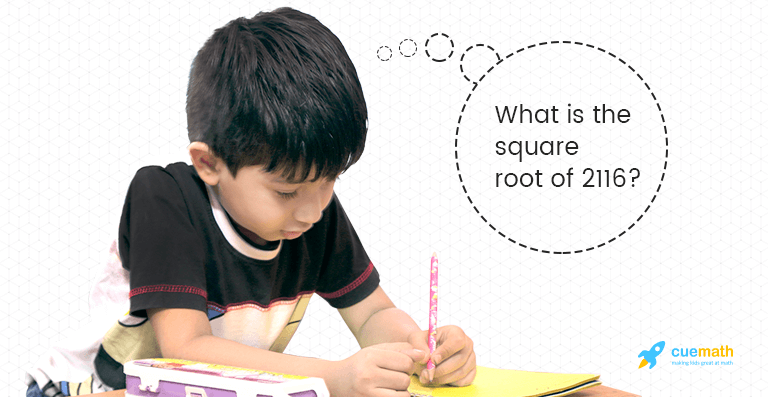# How To Estimate The Square Root Of A Perfect Square

Go back to  'Math Concepts'Squares and square roots imply multiplication and division algorithms. Students learn the squares by heart and make use of these in examinations. But do they know how squares are used in real life? As a teacher, it is your responsibility to help a student understand the importance of learning squares and square roots.

For example, Rhea is looking for an apartment in Delhi for rent. She is looking for a 2 bedroom apartment where she can live with her sister. A few phone calls later, she has shortlisted two places after seeing their pictures online. One is 1600 square feet with a balcony and the other is 2500 square feet with two balconies.

Now if Rhea knows her perfect squares, she can easily gauge the size of the first apartment as 40 feet by 40 feet. The second apartment would be 50 feet by 50 feet.

However, if she does not know how to break down 1600 and 2500, she would have to wait until she saw the place to decide if it was big enough for her.

What is a perfect square?

A perfect square is the product of an integer with itself. For example, 25 is the perfect square of 5 and -5.

5×5=25.

-5X-5 = 25

A square is always non-negative. However, the square root can be either a positive or a negative integer.

5×5 is usually denoted with 2 as a superscript as shown below.How to estimate the square root

Students are taught how to calculate square roots in class. What if we told you that you could teach them how to “estimate” square roots or rather large perfect squares?

The skill of estimating quantities happens only with practice. This does not mean that one should know how to calculate fast.

We start by knowing the squares of the first 10 natural numbers.From the above chart, we can conclude that a perfect square cannot have 2, 3, 7 or 8 in the unit’s digit.

A few other things to keep in mind:

1. If a perfect square ends with a 1, then the unit’s digit of its square root has to be either 1 or 9.
2. If a perfect square ends with a 4, then the unit’s digit of its square root has to be either 2 or 8.
3. If a perfect square ends with a 5, then the unit’s digit of its square root is definitely 5.
4. If a perfect square ends with a 6, then the unit’s digit of its square root has to be either 4 or 6.
5. If a perfect square ends with a 9, then the unit’s digit of its square root has to be either 3 or 7.

Now let’s try figure out the square root of 2116.

The first step is to determine how many digits the square root will have.

2116 has four digits, so the square root will have n/2 digits.

4/2=2 digits.

If a number has an odd number of digits ‘n’ then the square root will have (n+1)/2 digits.

We will divide 2116 into 2 groups. 21 and 16. (If we had taken a 3-digit number like 121, we would have considered 01 and 21.)

Let’s first work on the left most digits of the number, which is 21. The square that is closest and lesser than 21 is 16. The square root of 16 is 4.

So our first digit is 4.

The units digit of 2116 is 6. This would mean that the units digit of the square root is either 4 or 6.

Since the first digit of the square root is 4 and we know that it’s a two digit number, the square root of 2116 could either be 44 or 46. This lies between 40 and 50.

By squaring 40 and 50, we get 1600 and 2500 respectively. Now, let’s figure out which of these numbers is closer to 2116. This can be done by subtracting 21 from the first two digits of 1600 and 2500.

21-16= 5

25-21= 4

From this we can see that it’s closer to the square of 50.

Among 44 and 46, 46 is closer to 50.

Therefore, the square root of 2116 is 46.

Regular practice is needed to register this method in your mind. Once you get familiarized with this, estimating and finding out the square root of any perfect square would become quicker and much more easier.

Now try finding the square root of 3249 on your own.

Learn from the best math teachers and top your exams

• Live one on one classroom and doubt clearing
• Practice worksheets in and after class for conceptual clarity
• Personalized curriculum to keep up with school